## 2.7.1 Ballistic Transport

For ballistic transport one can consider an ideal case where electrons are not scattered on the wire of length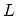connected to two electrodes,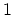and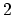(see Fig. 2.13). Since two electrodes have a large electron capacity, the FERMI energy for electrodesandare constants denoted by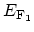and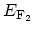, (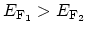), respectively. If there are no reflections of electrons at the electrodes,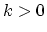states are occupied primarily by electrons coming from the left contact while the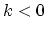states are occupied primarily by electrons coming from the right contact. Consequently the occupation factors for theandstates are given by the FERMI functions for the left and right contacts, respectively.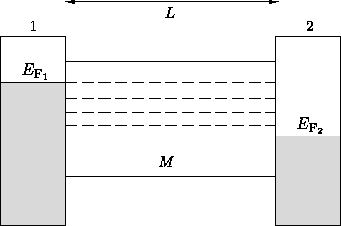Because of the confinement of electronic states in the direction perpendicular to the current flow, there are several energy subbands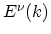. Thus the total current is given by the sum of the microscopic currents of all the subbands. The subbands are also called channels. The number of channels is a function of energy, which is denoted by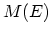. An electron which has a velocity of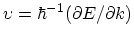in an unoccupied state contributes to the microscopic current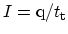, in which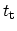is the carrier transit time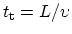. Then the total current is given by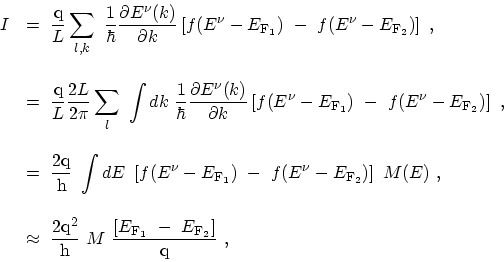(2.21)

where the sum over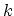is converted to the integral. In (2.21) a spin degeneracy ofand the inverse of the level spacing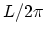is introduced andis assumed to be constant over the integration range. One can easily show that for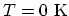only states with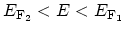contribute to the total current. If the width of a wire is very small (less than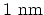),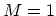even for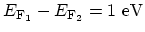. On the other hand, if the width of a wire is on the order of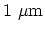and, the number of channels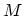becomes very large (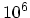).

Since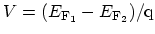is the voltage between the electrodes, the resistance of the ballistic conductor is given by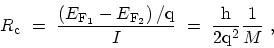(2.22)

where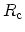is called the contact resistance and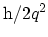is the quantized resistance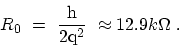(2.23)

This contact resistance arises from the mismatch of the numbers of conduction channels in the mesoscopic conductor and the macroscopic metal lead . In addition to this quantum-mechanical contact resistance, there are other sources of contact resistance, such as that produced by poor coupling between the mesoscopic conductor and the leads. The inverse of (2.22) gives the contact conductance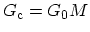where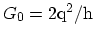denotes the quantized conductance. Thus in a wire without scattering the conductance is proportional to. The quantized resistance and conductance can be observed in clean semiconductors at very low temperature on samples which have a small number of channels. If one considers the range offor a SW-CNT with a diameter of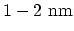, one finds thatunder low bias conditions,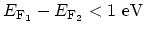. In a zigzag SW-CNT close to the FERMI energy the bands are doubly degenerate, and thus the total conductance is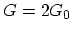.

In case of coherent transport, the wave-function is determined by the SCHRÖDINGER equation. The phase and amplitude of the wave-function at electrodecan be obtained from those at electrode. The resistance and the conductance are thus given by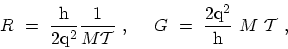(2.24)

where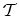is the transmission probability for a channel extending from electrodeto electrode. Here it is assumed again thatis constant near the FERMI energy. Equation (2.24) is known as the LANDAUER formula. It can be applied only if the wave-function spreads over the whole sample.

The resistance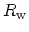for a single channel of a mesoscopic wire is given in terms of the transmission probabilityas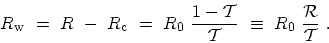(2.25)

The reflected wave-function, which is proportional to, causes a voltage drop in the wire.

M. Pourfath: Numerical Study of Quantum Transport in Carbon Nanotube-Based Transistors